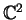suivant: Introduction

# A Bloch Constant for Hyperholomorphic Functions1

Dominic Rochon

June, 2000

Résumé
We use a commutative generalization of complex numbers called bicomplex numbers to show that the subclass of holomorphic mappings ofsatisfying the complexified Cauchy-Riemann equations has a Bloch constant in. Moreover, we find estimates when the mappings are on the unit ball and we give a specific domain ofwhere the Bloch constant has the same value as the classical Bloch constant for one variable.

Keywords: Bicomplex numbers, Bloch constant, Quasiregular mappings.
AMS subject classification: 30G, 30G35, 32A, 32A30.

Dominic Rochon
2000-07-26